#Function Repository Resource:

# InShuffle

Perform an in shuffle on a list

Contributed by: Sander Huisman
 ResourceFunction["InShuffle"][list] performs an in shuffle on list. ResourceFunction["InShuffle"][list,n] performs n in shuffles on list.

## Details and Options

An in shuffle is defined as follows: (1) split the list in two halves; and (2) interweave each half of the list starting with the second half, such that every other card came from the same half of the original list.
Here is an example of a list with 10 items: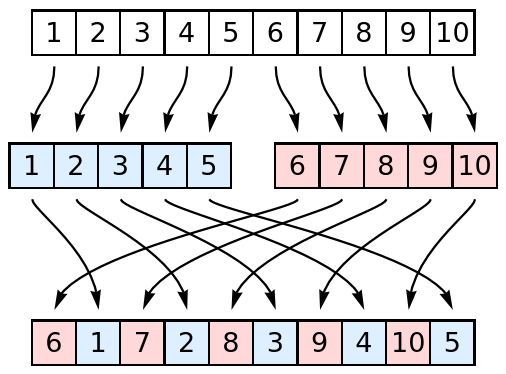When a list has an odd length n, the first "half" will be (n-1)/2 long and the second "half" will be (n+1)/2 long: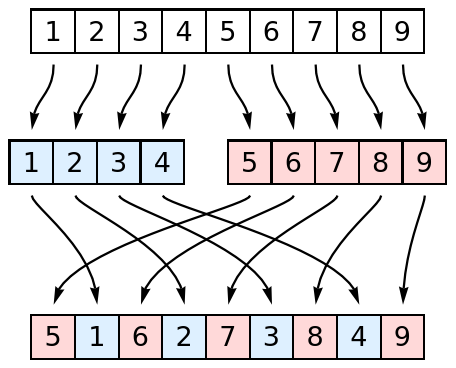## Examples

### Basic Examples

Perform an in shuffle on a list of 10 items:

 In:=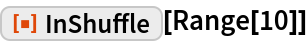Out=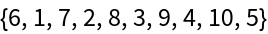### Scope

Perform an in shuffle 3 times to a list:

 In:=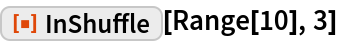Out=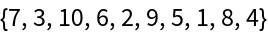Confirm that this is the same:

 In:=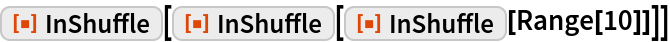Out=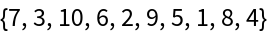The list can contain expressions of different type:

 In:=Out=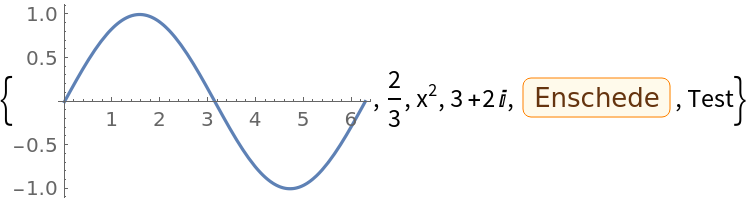### Applications

Create a sorted deck of cards:

 In:=Out=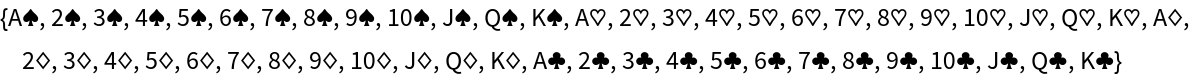Shuffle the deck once:

 In:=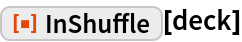Out=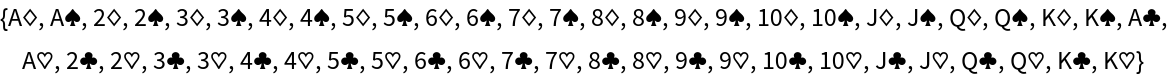The original is obtained after 52 shuffles:

 In:=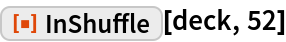Out=### Properties and Relations

An in shuffle on a list of length 2 can be seen as swapping the elements or reversing the list:

 In:=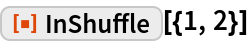Out=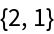Figure out after how many in shuffles a list of size n returns to its original order:

 In:=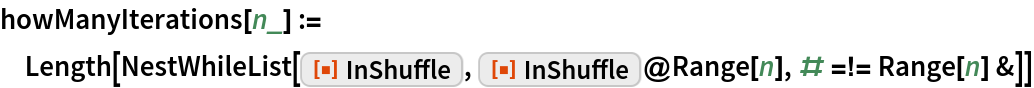For lists of even length, the number of shuffles to return to the original order is known analytically:

 In:=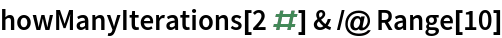Out=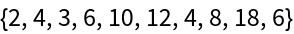In:=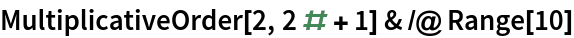Out=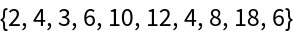Visualize how the positions of items are changed for a list of length 21 in the form of a Graph:

 In:=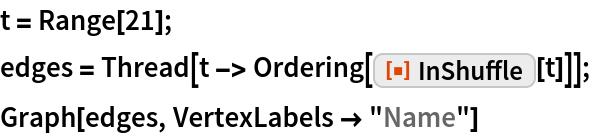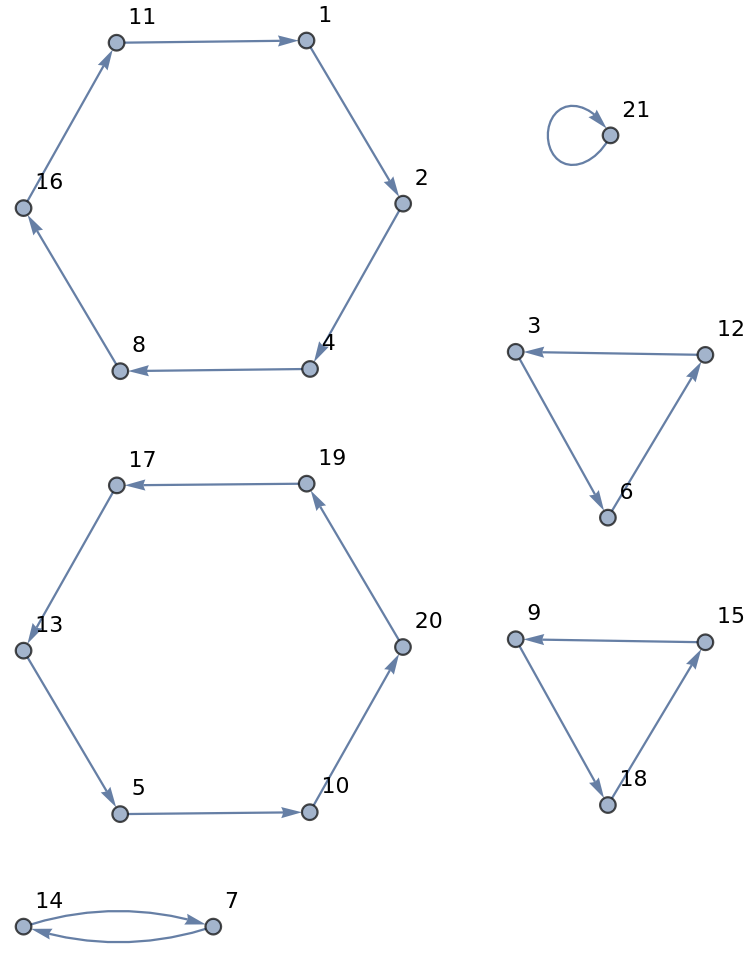Confirm that 6 nested in shuffles returns the list of 21 items to the original order:

 In:=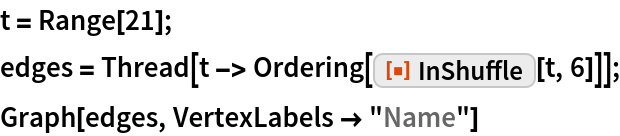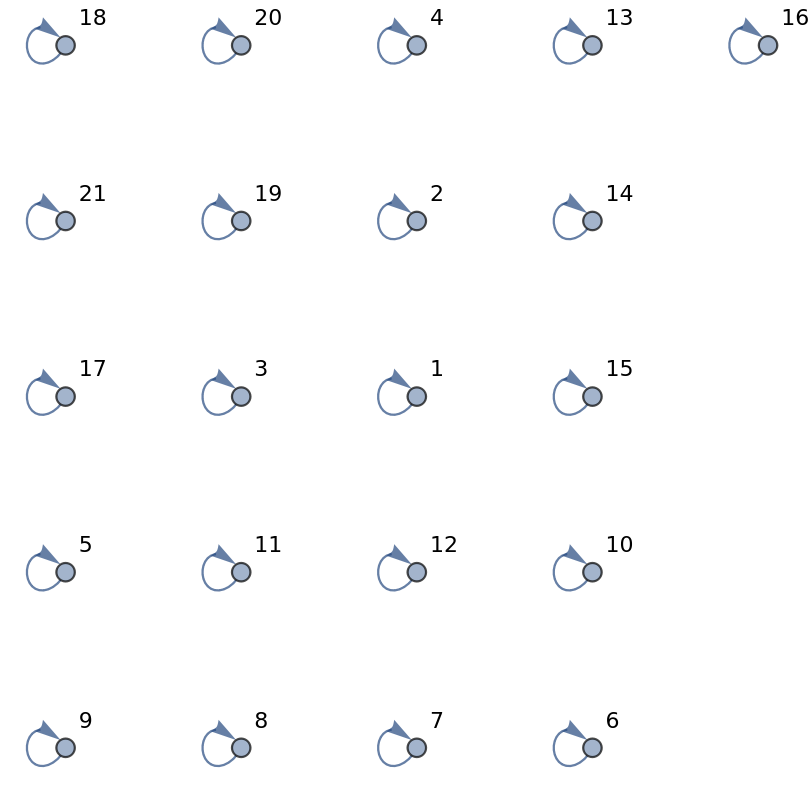### Possible Issues

Zero iterations returns the original list:

 In:=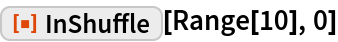Out=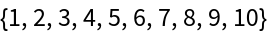### Neat Examples

Plot how many in shuffles are needed to return to the original order for a list of length n:

 In:=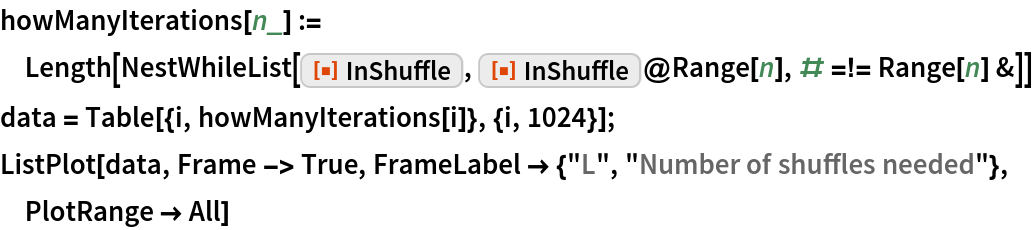Out=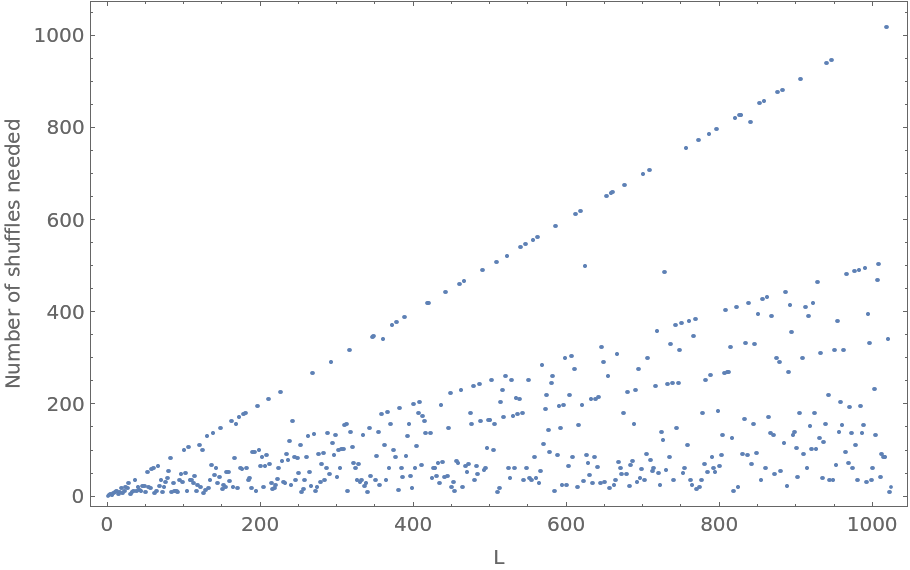## Requirements

Wolfram Language 11.3 (March 2018) or above